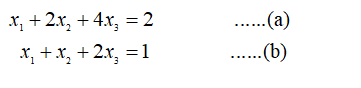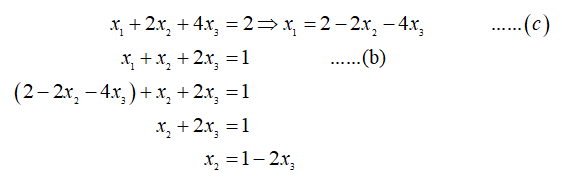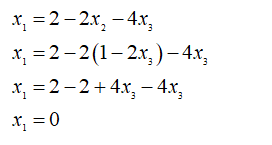# Solve the following system of equations. (Enter your answers as a comma-separated list. If there are infinitely many solutions, enter a parametric solution using t and/or s. If there is no solution, enter NONE.)x1 + 2x2 + 4x3 = 2x1 + x2 + 2x3 = 1(x1, x2, x3) =

Question
2 views

Solve the following system of equations. (Enter your answers as a comma-separated list. If there are infinitely many solutions, enter a parametric solution using t and/or s. If there is no solution, enter NONE.)

 x1 + 2x2 + 4x3 = 2 x1 + x2 + 2x3 = 1
(x1, x2, x3) =

check_circle

Step 1

Here the given equations are:Step 2

Isolated x1 from equation (a) then substitute that value in equation (b):Step 3

Now, put the value of x2  in equation (c) :...

### Want to see the full answer?

See Solution

#### Want to see this answer and more?

Solutions are written by subject experts who are available 24/7. Questions are typically answered within 1 hour.*

See Solution
*Response times may vary by subject and question.
Tagged in

### Algebra